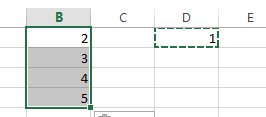# How to Convert Text to Number in Excel

This post will guide you how to convert text or letters to number in Excel. How do I convert text to number with a formula in Excel. How to convert text-based numbers to numeric value in Excel.

Assuming that you have a list of data in range B1:B4, which contain some numbers stored as text in your worksheet. And you need to convert those text-based numbers to numeric value for calculation when using SUM or other Functions. Here are the methods that you can use to convert text into a number.

## Convert Text to Number with a Formula

If you want to convert text values to numbers, you can use a formula based on the VALUE function. Or you can also add zero for text values. The formula is as below:

=VALUE(B1)

Or

=B1 +0

Type this formula into a blank cell and press Enter key on your keyboard. And then drag the AutoFill handle over to other cells to apply this formula.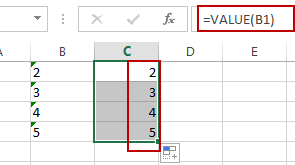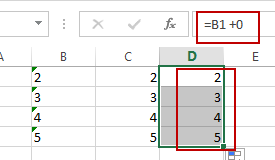Note: The VALUE function will try to convert a number stored as text to a true number. So this formula will return a numeric value.

## Convert Text to Number with Convert to Number Option

When you add one apostrophe into a number, and it will convert the number formula as text format. And you should notice that there is a green triangle at the top left part of the cell.

If you want to convert text to number again, just do the following steps:

#1 select the range of cells that you want to convert.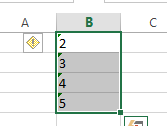#2 click on the yellow diamond shape icon, and select Convert to Number from the drop down menu list.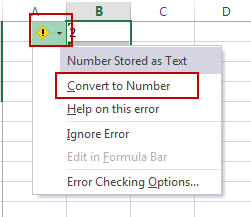You should see that all text values in the selected range have been converted to numbers back.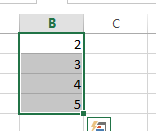## Convert Text to Number with Format Cell Function

If the numbers are formatted as text format, and you can also use the Format Cell function to convert test format to number format easily. Just do the following steps:

#1 select the range of cells that you want to convert.#2 right click on it, and select Format Cell from the drop down menu list. And the Format Cells dialog will open.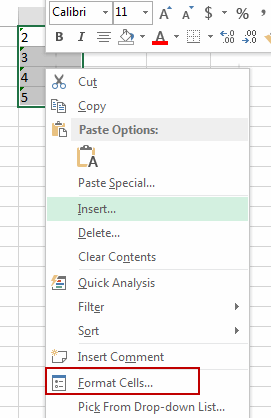#3 Select “Number” tab, then you can select Number category in the category ListBox. The formatting result will be appear on the “Sample” section on the “Format Cells” window. Click Ok button.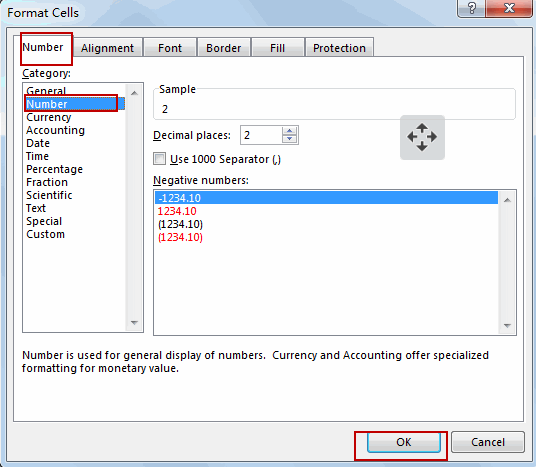Or you can go to HOME tab, click Number command, and select General from the Number Format drop down list.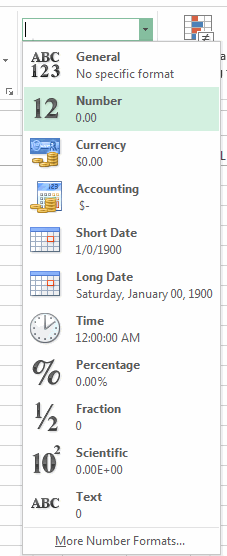This would change the format of the selected cells to general numbers.  And you can also change the number category to other formats, such as: Currency, Accounting, etc.

## Convert Text to Number with Paste Special

You can also use Paste Special Option to achieve the same result of converting text to numbers in Excel. Here are the steps:

#1 Enter number 1 in an empty cell in your worksheet, and you should make sure that it is formatted as number. And press Ctrl + C to copy this cell that contains 1.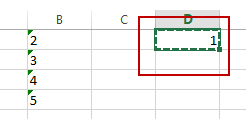#2 select the range of cells that you want to convert its to numbers.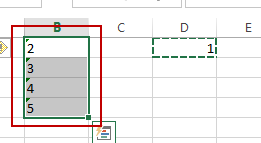#3 right click on it, and select Paste Special from the popup menu list.  And the Paste Special dialog will appear.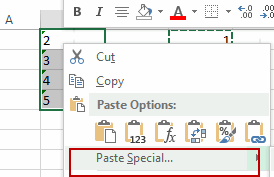#4 select Multiply radio button under Operation section. And click Ok button.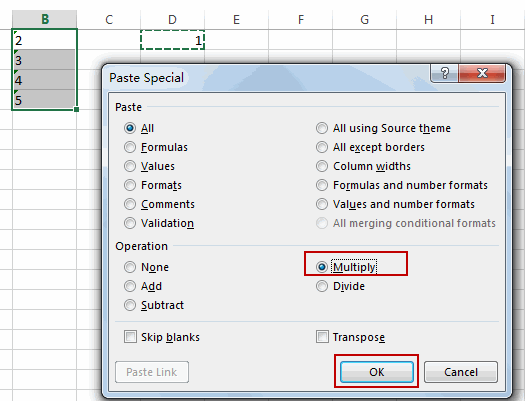#5  you would notice that the text values in the selected range have been converted to numbers.